# Binary search tree insertion

• Adding a new node in the binary tree should not violate the properties of the binary tree
• the root value is checked with the root node
• case 1: If the new node value is less than the root value traversing should be done left subtree.
• case 2: If the new node value is greater than the root value traversing should be done right subtree.
• The search pointer moves down until it reaches the leaf node. the insertion function requires time proportional to the height of the tree.in the most case, it takes o(log n) time to execute in the average it lakes o(n)

lets look into one example:

#### insert 41 in the below binary tree#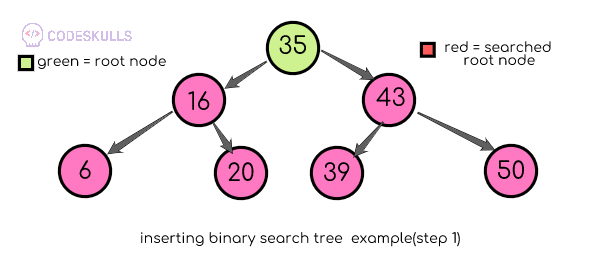step 1: compare 41 with the root node 35. (41>35)41 is greater than 35 so traversal should be done on the right subtree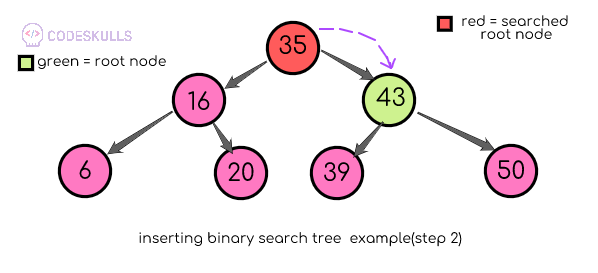step 2:compare 41 with root node 43. (41<43) 41 is lesser than 43 so traversal should be done on the left sub tree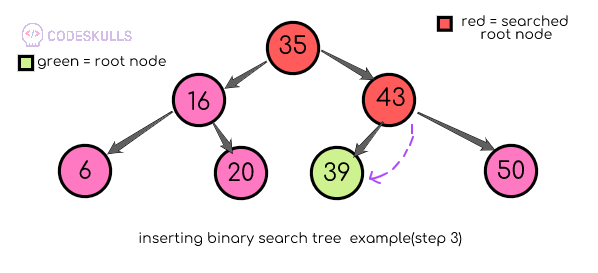step 3: compare 41 with root node 39. (41>39) 41 is greater than 39 so traversal should be done on the right subtree, but there is no sub-nodes for the root node, a new node should be inserted as a right subtree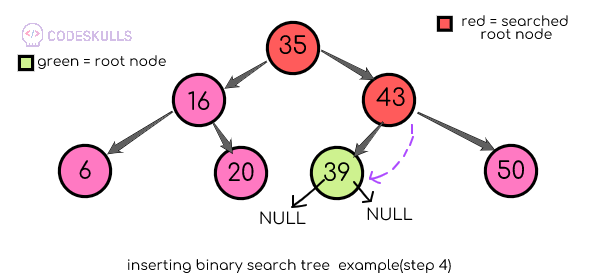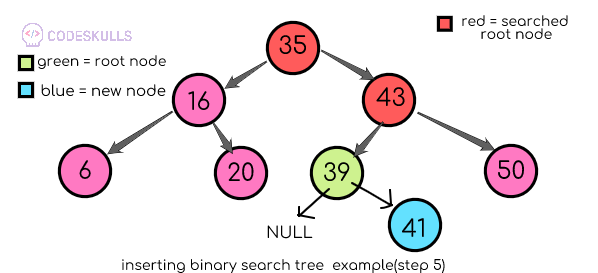Last updated on by vishal devxo